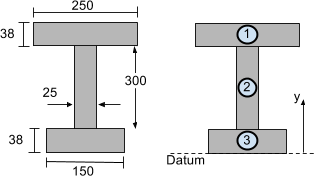# Calculate the Centroid of a Beam Section

## How to Calculate the Centroid of a Beam Section

The centroid or center of mass of beam sections is useful for beam analysis when the moment of inertia is required for calculations such as shear/bending stress and deflection. Beam sections are usually made up of one or more shapes. So to find the centroid of an entire beam section area, it first needs to be split into appropriate segments. After this, the area and centroid of each individual segment need to be considered to find the centroid of the entire section. Alternatively, try our free Centroid Calculator

Consider the I-beam section shown below. To calculate the vertical centroid (in the y-direction) it can be split into 3 segments as illustrated:Now we simply need to use the formula for calculating the vertical (y) centroid of a multi-segment shape:

[math] \bar{y}=\frac{\sum{A}_{i}{y}_{i}}{\sum{A}_{i}}\text{ where:}\\\\ \begin{align} {A}_{i} &= \text{The individual segment's area}\\ {y}_{i} &= \text{The individual segment's centroid distance from a reference line or datum} \end{align} [math]

We will take the datum or reference line from the bottom fo the beam section. Now let's find Ai and yi for each segment of the I-beam section shown above so that the vertical or y centroid can be found.

[math] \text{Segment 1:}\\ \begin{align} {A}_{1} &= 250\times38 = 9500 {\text{ mm}}^{2}\\ {y}_{1} &= 38 + 300 + \tfrac{38}{2} = 357 \text{ mm}\\\\ \end{align} [math] [math] \text{Segment 2:}\\ \begin{align} {A}_{2} &= 300\times25 = 7500 {\text{ mm}}^{2}\\ {y}_{2} &= 38 + \tfrac{300}{2} = 188 \text{ mm}\\\\ \end{align} [math] [math] \text{Segment 3:}\\ \begin{align} {A}_{3} &= 38\times150 = 5700 {\text{ mm}}^{2}\\ {y}_{3} &= \tfrac{38}{2} =19 \text{ mm}\\\\ \end{align} [math] [math] \begin{align} \therefore \bar{y} &=\frac{\sum{y}_{i}{A}_{i}}{\sum{A}_{i}}\\ &=\frac{{A}_{1}{y}_{1} + {A}_{2}{y}_{2} + {A}_{3}{y}_{3}}{{A}_{1} + {A}_{2} + {A}_{3}}\\ &=\frac{(9500\times357) + (7500\times188) + (5700\times19)}{9500 + 7500 + 5700}\\ \bar{y} &=216.29\text{ mm} \end{align} [math]

In the event that the cross-section is composed of two materials or a composite material, then one of the materials will have to be multiplied by the modular ratio n such that the equation entire section becomes uniform.

[math] n=\frac{E_{1}}{E_{2}} [math]

Typically, E1 is the modulus of elasticity the non-prevailing material and E2 is the modulus of elasticity of the prevailing material, although whichever order that is preferred will not affect the solution of the centroid. Adjusting for the second material, the formula for the centroid becomes the following.

[math] \bar{y}=\frac{\sum{A}_{i}{y}_{i}+\sum {n}{A}_{i}{y}_{i}}{\sum{A}_{i}+\sum {n}{A}_{i}} [math]

Of course, manually computing for the moment of inertia is not necessary with the use of our fantastic Centroid Calculator to find the vertical (y) and horizontal (x) centroids of beam sections.

For more complete list of sections and functionality, you can try out our Section Builder

Visit the next step: How to Calculate the Moment of Inertia of a Beam Section.